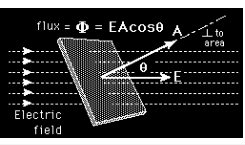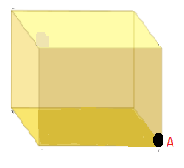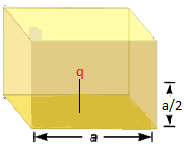# MCQ on Electric flux

The total number of electric field lines passing a given area in a unit time is defined as the electric flux.If the plane is normal to the flow of the electric field, the total flux is given as:

Φ=EA

When the same plane is tilted at an angle Ɵ, the projected area is given as AcosƟ and the total flux through this surface is given as

Φ = EACosθ

Where,

• E is the magnitude of the electric field
• A is the area of the surface through which the electric flux is to be calculated
• Ɵ is the angle made by the plane and the axis parallel to the direction of flow of the electric field

Q1: What is the value of total flux through the faces of the cube with the sides of length a if a charge Q is placed at corner A of the cube is1. q/ε0
2. q/2ε0
3. q/4ε0
4. q/8ε0

Q2: If a unit positive charge is kept in the air. Then the total flux coming out of unit charge is

1. 4πε0-1
2. 4πε0
3. ε0-1
4. ε0

Q3: What is the value of electric flux (Φ) on a plane of area 1m2 on which an electric field of 2 V/m crosses with an angle of 300.

1. 1 Vm
2. 2 Vm
3. 3 Vm
4. 4 Vm

Q4: Determine the electric flux of a flat square having an area of 10m2 is a uniform electric field of 8000 N/C passing perpendicular to it

1. 8 x 105 Nm2/C
2. 8 x 104 Nm2/C
3. 16 x 105 Nm2/C
4. 4 x 104 Nm2/C

Answer: (b) 8 x 104 Nm2/C

Q5: A plane surface is rotated in a uniform electric field. When is the flux of the electric field through the surface maximum?

1. When the surface is perpendicular to the field
2. When the surface is parallel to the field
3. When the surface is at an angle of 300 with the field
4. When the surface is at an angle of 450 with the field

Answer: (a) When the surface is perpendicular to the field

Q6: When is the flux through a surface taken as positive

1. When the flux lines are directed inwards
2. When the flux lines are directed outwards
3. No flux lines through the surface
4. Flux lines are parallel to each other

Answer: (b) When the flux lines are directed outwards

Q7: The net charge through a closed surface in a given medium depends on

1. Size of the surface
2. Charge of the surface
3. The shape of the surface
4. Area of the surface

Answer: (b) Charge of the surface

Q8: The dimension of electric flux is

1. [M2L2T3A1]
2. [MLT-3A-1]
3. [M3L3T-3A-1]
4. [ML3T -3A-1]

Q9: The electric flux through a cubical Gaussian surface enclosing net charge q is q/ε0. While the electric flux through one face of a cube is

1. q/ε0
2. q/6ε0
3. q/4πε0
4. q/4ε0

Q10: A point charge q is placed at a distance a/2 directly above the centre of the square of side a. The electric flux through the square is

1. q/ε0
2. q/6ε0
3. q/4πε0
4. q/4ε0

Explanation for Q10: Imagine a cube with charge q at the centre and the given square is one of its faces. So electric flux through the given square( one face) q/6ε0Explore more:

1. laiba khan